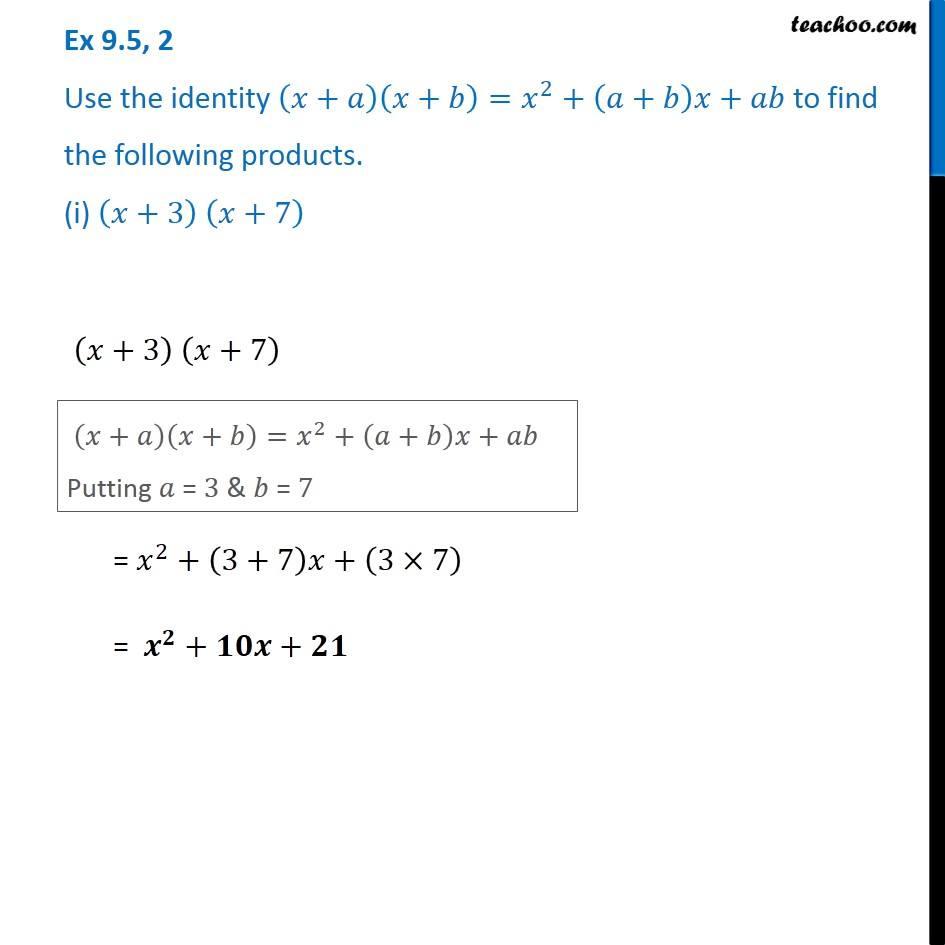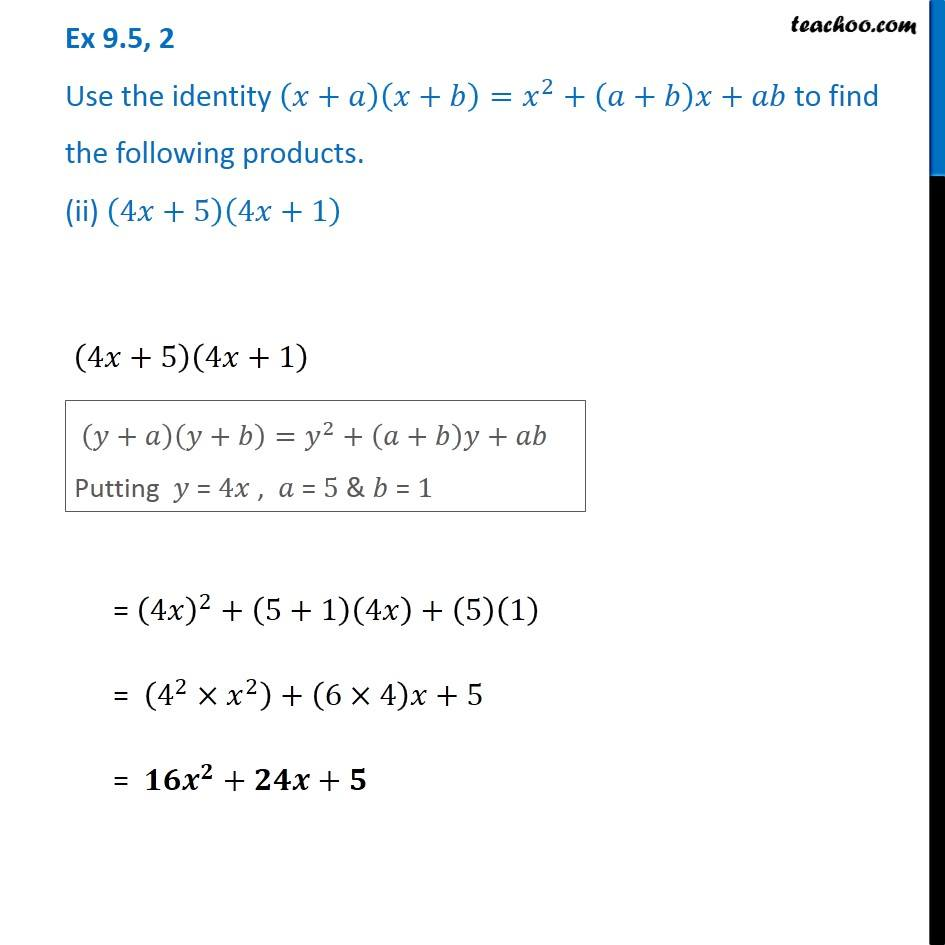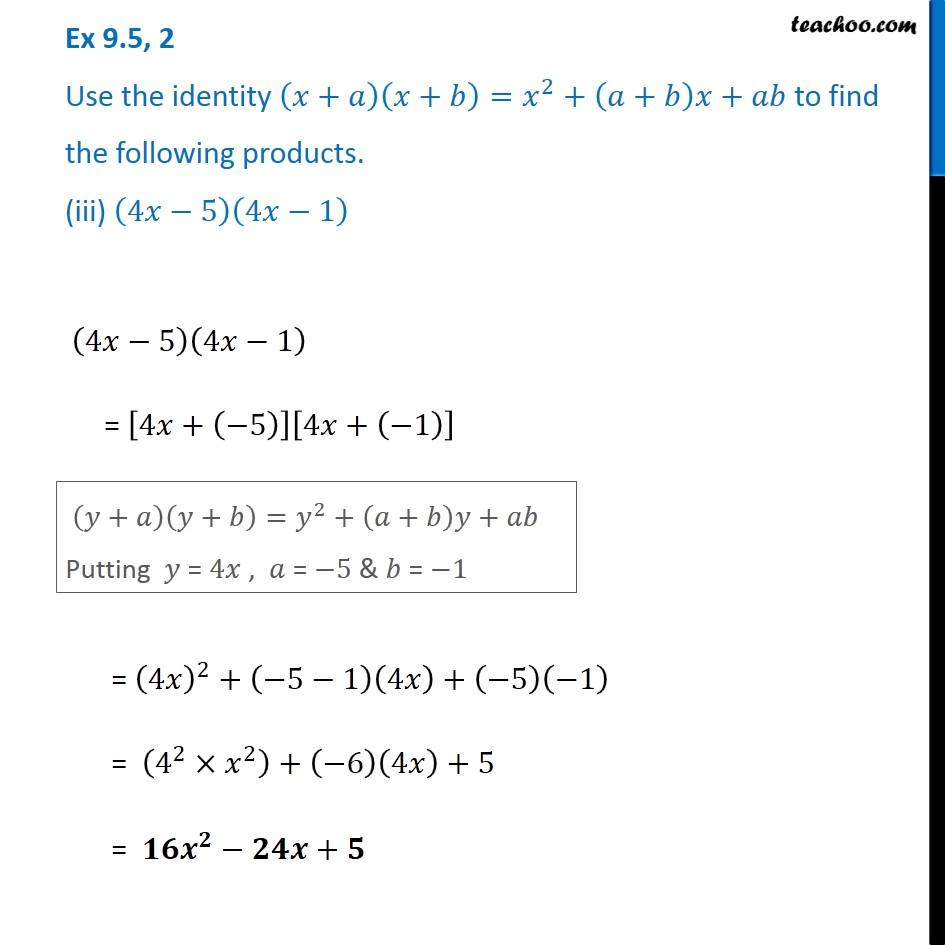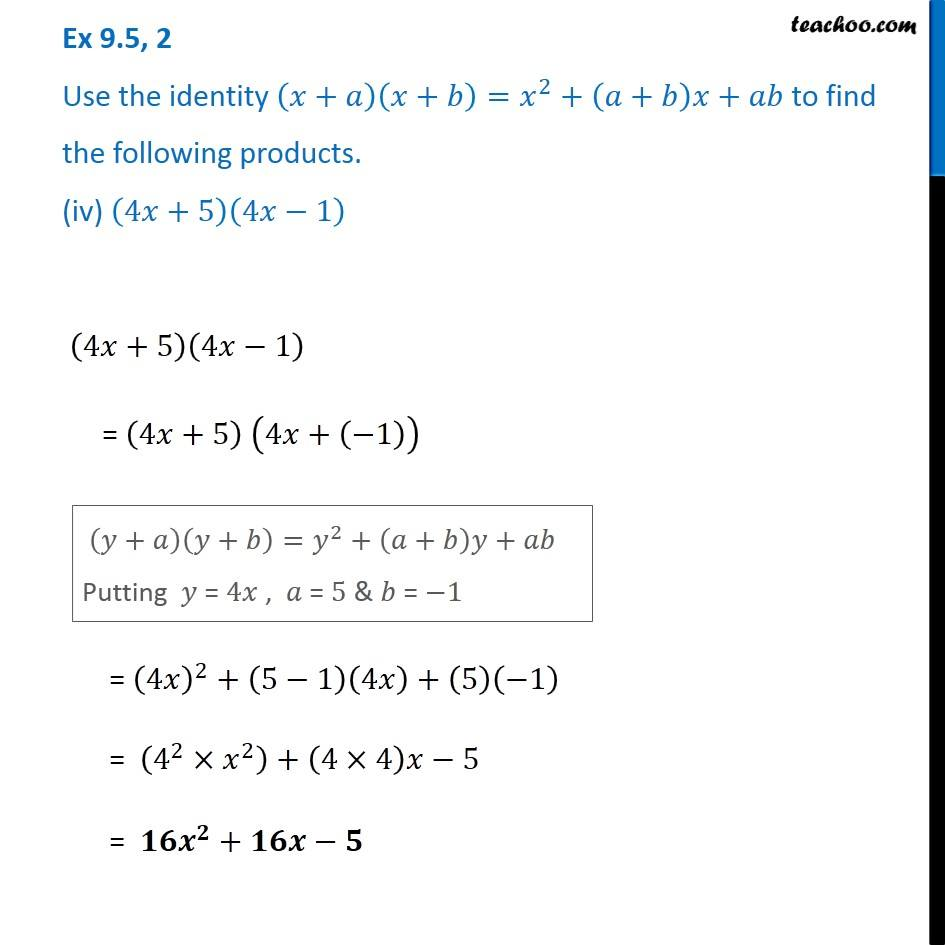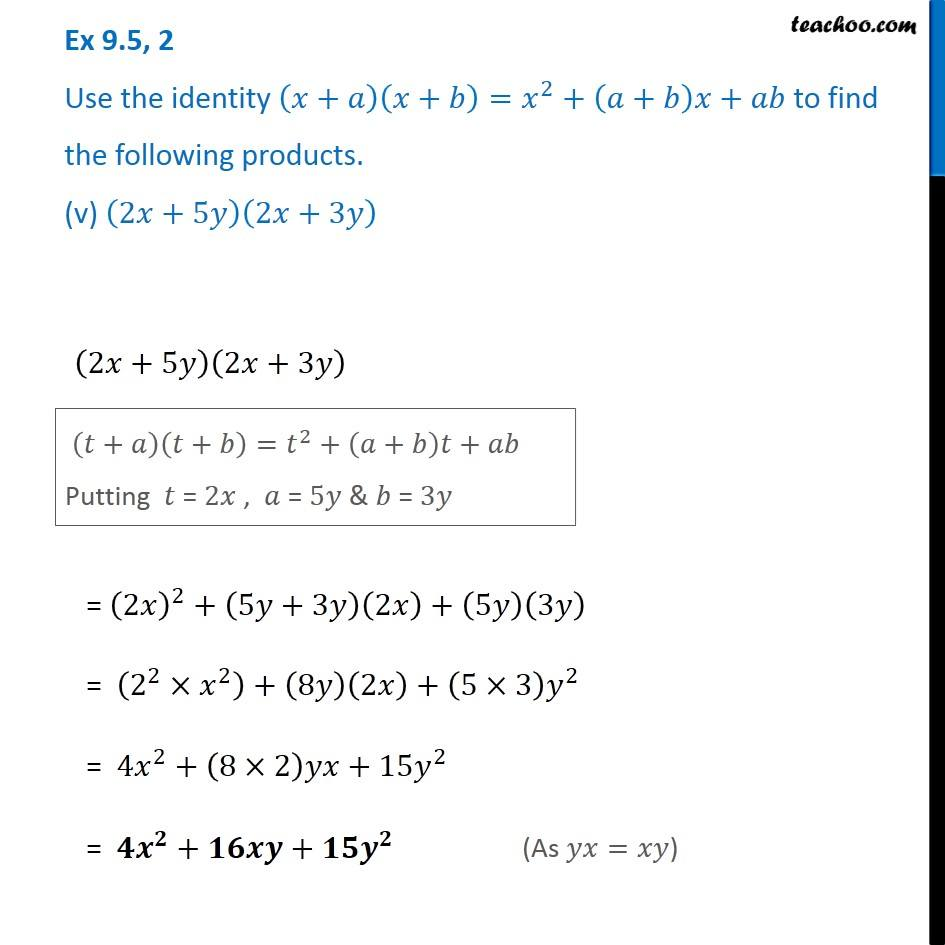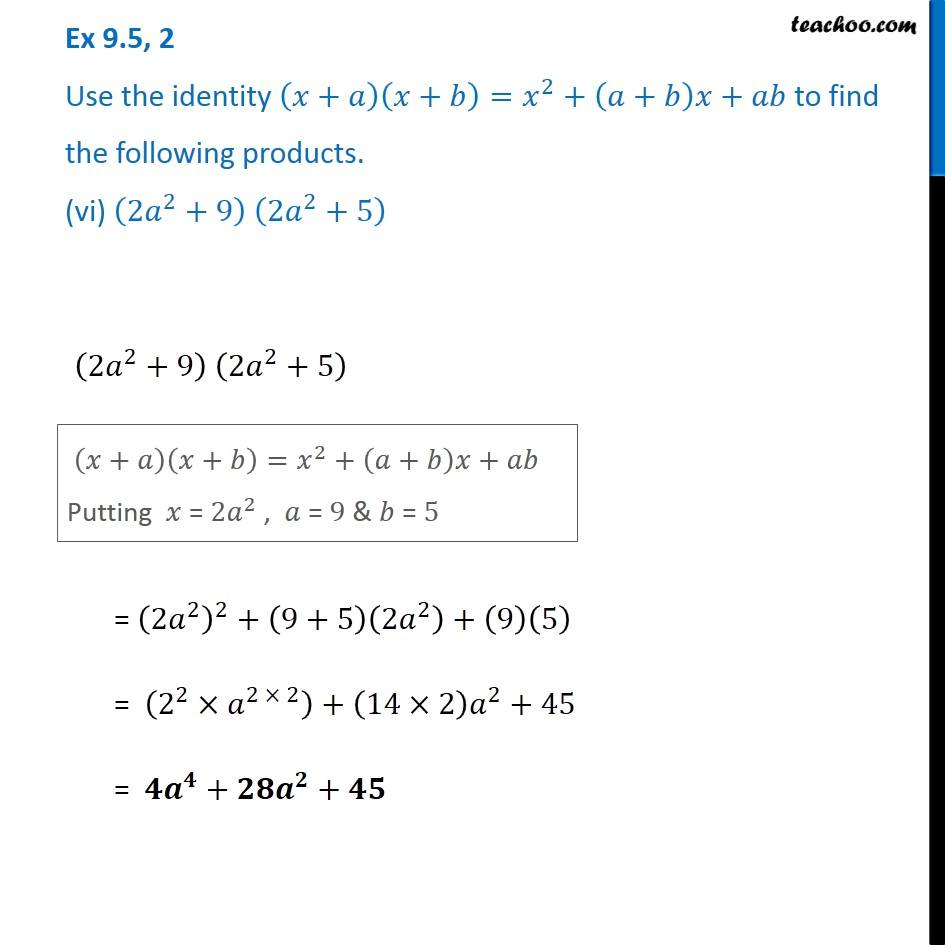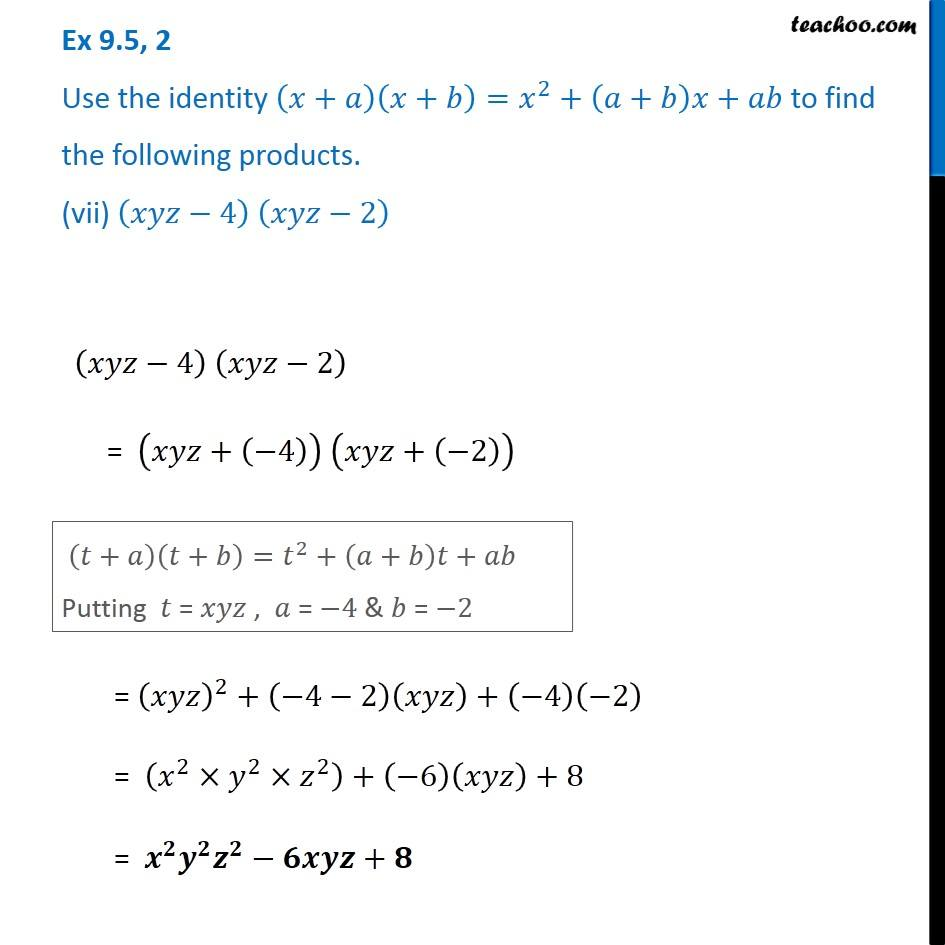1. Chapter 9 Class 8 Algebraic Expressions and Identities
2. Serial order wise
3. Ex 9.5

Transcript

Ex 9.5, 2 Use the identity (𝑥+𝑎)(𝑥+𝑏)=𝑥^2+(𝑎+𝑏)𝑥+𝑎𝑏 to find the following products. (i) (𝑥+3) (𝑥+7) (𝑥+3) (𝑥+7) (𝑥+𝑎)(𝑥+𝑏)=𝑥^2+(𝑎+𝑏)𝑥+𝑎𝑏 Putting 𝑎 = 3 & 𝑏 = 7 = 𝑥^2+(3+7)𝑥+(3×7) = 𝒙^𝟐+𝟏𝟎𝒙+𝟐𝟏 Ex 9.5, 2 Use the identity (𝑥+𝑎)(𝑥+𝑏)=𝑥^2+(𝑎+𝑏)𝑥+𝑎𝑏 to find the following products. (ii) (4𝑥+5)(4𝑥+1) (4𝑥+5)(4𝑥+1) (𝑦+𝑎)(𝑦+𝑏)=𝑦^2+(𝑎+𝑏)𝑦+𝑎𝑏 Putting 𝑦 = 4𝑥 , 𝑎 = 5 & 𝑏 = 1 = (4𝑥)^2+(5+1)(4𝑥)+(5)(1) = (4^2×𝑥^2 )+(6×4)𝑥+5 = 𝟏𝟔𝒙^𝟐+𝟐𝟒𝒙+𝟓 Ex 9.5, 2 Use the identity (𝑥+𝑎)(𝑥+𝑏)=𝑥^2+(𝑎+𝑏)𝑥+𝑎𝑏 to find the following products. (iii) (4𝑥−5)(4𝑥−1) (4𝑥−5)(4𝑥−1) = [4𝑥+(−5)][4𝑥+(−1)] (𝑦+𝑎)(𝑦+𝑏)=𝑦^2+(𝑎+𝑏)𝑦+𝑎𝑏 Putting 𝑦 = 4𝑥 , 𝑎 = −5 & 𝑏 = −1 = (4𝑥)^2+(−5−1)(4𝑥)+(−5)(−1) = (4^2×𝑥^2 )+(−6)(4𝑥)+5 = 𝟏𝟔𝒙^𝟐−𝟐𝟒𝒙+𝟓 Ex 9.5, 2 Use the identity (𝑥+𝑎)(𝑥+𝑏)=𝑥^2+(𝑎+𝑏)𝑥+𝑎𝑏 to find the following products. (iv) (4𝑥+5)(4𝑥−1) (4𝑥+5)(4𝑥−1) = (4𝑥+5) (4𝑥+(−1)) (𝑦+𝑎)(𝑦+𝑏)=𝑦^2+(𝑎+𝑏)𝑦+𝑎𝑏 Putting 𝑦 = 4𝑥 , 𝑎 = 5 & 𝑏 = −1 (𝑦+𝑎)(𝑦+𝑏)=𝑦^2+(𝑎+𝑏)𝑦+𝑎𝑏 Putting 𝑦 = 4𝑥 , 𝑎 = 5 & 𝑏 = −1 Ex 9.5, 2 Use the identity (𝑥+𝑎)(𝑥+𝑏)=𝑥^2+(𝑎+𝑏)𝑥+𝑎𝑏 to find the following products. (v) (2𝑥+5𝑦)(2𝑥+3𝑦) (2𝑥+5𝑦)(2𝑥+3𝑦) (𝑡+𝑎)(𝑡+𝑏)=𝑡^2+(𝑎+𝑏)𝑡+𝑎𝑏 Putting 𝑡 = 2𝑥 , 𝑎 = 5𝑦 & 𝑏 = 3𝑦 (𝑡+𝑎)(𝑡+𝑏)=𝑡^2+(𝑎+𝑏)𝑡+𝑎𝑏 Putting 𝑡 = 2𝑥 , 𝑎 = 5𝑦 & 𝑏 = 3𝑦 (As 𝑦𝑥=𝑥𝑦) Ex 9.5, 2 Use the identity (𝑥+𝑎)(𝑥+𝑏)=𝑥^2+(𝑎+𝑏)𝑥+𝑎𝑏 to find the following products. (vi) (2𝑎^2+9) (2𝑎^2+5) (2𝑎^2+9) (2𝑎^2+5) (𝑥+𝑎)(𝑥+𝑏)=𝑥^2+(𝑎+𝑏)𝑥+𝑎𝑏 Putting 𝑥 = 2𝑎^2 , 𝑎 = 9 & 𝑏 = 5 = (2𝑎^2 )^2+(9+5)(2𝑎^2 )+(9)(5) = (2^2×𝑎^(2 × 2) )+(14×2) 𝑎^2+45 = 𝟒𝒂^𝟒+𝟐𝟖𝒂^𝟐+𝟒𝟓 Ex 9.5, 2 Use the identity (𝑥+𝑎)(𝑥+𝑏)=𝑥^2+(𝑎+𝑏)𝑥+𝑎𝑏 to find the following products. (vii) (𝑥𝑦𝑧−4) (𝑥𝑦𝑧−2) (𝑥𝑦𝑧−4) (𝑥𝑦𝑧−2) = (𝑥𝑦𝑧+(−4)) (𝑥𝑦𝑧+(−2)) (𝑡+𝑎)(𝑡+𝑏)=𝑡^2+(𝑎+𝑏)𝑡+𝑎𝑏 Putting 𝑡 = 𝑥𝑦𝑧 , 𝑎 = −4 & 𝑏 = −2 = (𝑏)^2+(7)^2−2(𝑏)(7) = 𝑏^2+49−(2×7)×𝑏 = 𝒃^𝟐+𝟒𝟗−𝟏𝟒𝒃

Ex 9.5